# Calculator addition of two mixed numbers

The calculator performs basic and advanced operations with mixed numbers, fractions, integers, decimals. Mixed fractions are also called mixed numbers. A mixed fraction is a whole number and a proper fraction combined, i.e. one and three-quarters. The calculator evaluates the expression or solves the equation with step-by-step calculation progress information. Solve problems with two or more mixed numbers fractions in one expression.

### 21/2 + 42/3 = 43/6 = 7 1/6 ≅ 7.1666667

Spelled result in words is seven and one sixth (or forty-three sixths).

### Calculation steps

1. Conversion a mixed number 2 1/2 to a improper fraction: 2 1/2 = 2 1/2 = 2 · 2 + 1/2 = 4 + 1/2 = 5/2

To find a new numerator:
a) Multiply the whole number 2 by the denominator 2. Whole number 2 equally 2 * 2/2 = 4/2
b) Add the answer from previous step 4 to the numerator 1. New numerator is 4 + 1 = 5
c) Write a previous answer (new numerator 5) over the denominator 2.

Two and one half is five halfs
2. Conversion a mixed number 4 2/3 to a improper fraction: 4 2/3 = 4 2/3 = 4 · 3 + 2/3 = 12 + 2/3 = 14/3

To find a new numerator:
a) Multiply the whole number 4 by the denominator 3. Whole number 4 equally 4 * 3/3 = 12/3
b) Add the answer from previous step 12 to the numerator 2. New numerator is 12 + 2 = 14
c) Write a previous answer (new numerator 14) over the denominator 3.

Four and two thirds is fourteen thirds
3. Add: 5/2 + 14/3 = 5 · 3/2 · 3 + 14 · 2/3 · 2 = 15/6 + 28/6 = 15 + 28/6 = 43/6
For adding, subtracting, and comparing fractions, it is suitable to adjust both fractions to a common (equal, identical) denominator. The common denominator you can calculate as the least common multiple of both denominators - LCM(2, 3) = 6. In practice, it is enough to find the common denominator (not necessarily the lowest) by multiplying the denominators: 2 × 3 = 6. In the following intermediate step, the fraction result cannot be further simplified by canceling.
In other words - five halfs plus fourteen thirds = forty-three sixths.

## Mixed fractions in word problems:

• A science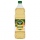A science experiment calls for making 3 and 2/3 cups of distilled water with 1 and 3/5 cups of vinegar and 2/3 cups of liquid detergent. How much liquid in all and cups is needed?
• A more than bWhat is 5 1/7 more than 2 3/7?Add this two mixed numbers: 1 5/6 + 2 2/11=Add this two mixed numbers: 1 5/6 + 2 2/11=
• Team runThe first team member in a 926-person relay race must run 2 1/4 laps, the second team member must run 1 1/2 laps, and the third team member must run 3 1/4 laps. How many laps in all must each team run?Add two mixed fractions: 2 4/6 + 1 3/62 and 1/8th plus 1 and 1/3rd =
• Sum of 20Sum of the two numbers is 15 1/6. If one of the numbers is 2 2/3, find the other number.
• Zailene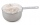Zailene wants to make two kinds of kakanin, puto and kutsina. Puto recipe needs 3 2/5 cup of flour while Kutsinta needs 5 ¼ cup of flour. How many cups of flour will Zailene be needed in all?3 3/4 + 2 3/5 + 5 1/2 Show your solution.
• Jack planted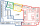Jack planted 1 1/4 rows of lettuce in the family garden, Rose planted 1 1/2 rows of broccoli and Calvin planted 4 5/12 rows of beans. How many rows were planted in the garden?
• Donuts 3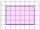You pick up donuts for a party. You have 3 and 4/12 boxes of donuts. Roy picks up donuts too. He has 2 and 9/12 boxes of donuts. How many boxes of donuts do you have in all?
• Sum of 18Sum of two fractions is 4 3/7. If one of the fractions is 2 1/5 find the other one .
• Sum of mixedThe sum of two mixed numbers is 15½. One of the number is 5 ¾, what is the other?
• Dog food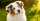A whole bag of dog food weighs 5 1/2 pounds. How much dog food do you have if there are 3 3/4 bags in the cupboard?
• Honey 3Honey jogged 1 3/4 km on Monday,1 1/2 km on Wednesday and 1  2/3 km on Friday . how far did he jog?
• Cloth / textile 2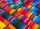4 and 3/10 yards of red cloth. 3 and 1/10 yards of pink cloth and 9 and 2/10 yards of black cloth. How many meters of cloth did she buy in all?